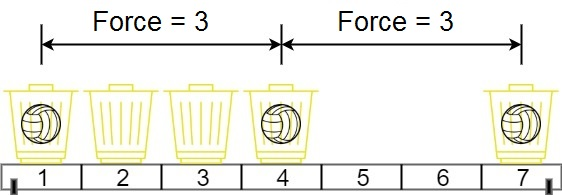# 两球之间的磁力

## 1552. 两球之间的磁力 (Medium)```输入：position = [1,2,3,4,7], m = 3

```

```输入：position = [5,4,3,2,1,1000000000], m = 2

```

• `n == position.length`
• `2 <= n <= 10^5`
• `1 <= position[i] <= 10^9`
• 所有 `position` 中的整数 互不相同 。
• `2 <= m <= position.length`

[数组] [二分查找] [排序]